# Inverse Engineer a RLC circuit from a transferfunction

#### Fredje

Joined Jun 1, 2022
19
I have an assignment for school where I need to design a RLC network of a given transferfunction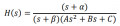It is a third order so I need 3 frequency dependend components. I need 3 poles and 1 zero. But how would I need to design a circuit given these recuirements? I tried a lot of different designs but never succeeded to get the correct transferfunction (mostly I ain't get a s in the numerator)

How could I get an extra zero in a RLC network?

#### Papabravo

Joined Feb 24, 2006
20,404
This problem is not "inverse engineering". It belongs to a class of problems called synthesis. That is: "How do I synthesize a circuit from a transfer function"

What you do is design the circuits individually and cascade them.

$$H(s)\;=\;C(s)G(s)$$
$$C(s)\;=\;\cfrac{(s+\alpha)}{(s+\beta)}$$
$$G(s)\;=\;\cfrac{1}{As^2+Bs+C}$$

For the transfer function C(s), google "Lead Lag Networks". When you cascade things like this you need to ensure that later stages do nor load prior stages or your design will not perform as expected. Unity gain buffers are often used for this purpose.

As this is a third order system you need to ensure that it is stable by doing a root locus, or some alternate method.

Last edited:
•Fredje

#### Fredje

Joined Jun 1, 2022
19

With the information you gave I cascaded a 'lead lag network' with a lowpass filter and a voltage follower in the middle.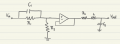I calculated the transferfunction and I indeed got a correct answer so thank you very much, you helped me a lot with this!

I just got some more questions so I can understand better what is happening.
- What is the buffer exactly doing. does it make sure the output impedance is ideal?
- Is it always needed to put a buffer between the two circuits, or is it also possible to design this RLC network without an active component in it?

#### Papabravo

Joined Feb 24, 2006
20,404

With the information you gave I cascaded a 'lead lag network' with a lowpass filter and a voltage follower in the middle.

View attachment 270319

I calculated the transferfunction and I indeed got a correct answer so thank you very much, you helped me a lot with this!

I just got some more questions so I can understand better what is happening.
- What is the buffer exactly doing. does it make sure the output impedance is ideal?
- Is it always needed to put a buffer between the two circuits, or is it also possible to design this RLC network without an active component in it?
The buffer has a high input impedance, so it presents a very small load to the first stage. It also has a low output impedance so most of the output voltage will appear across the output network. When you become more familiar with opamp circuits you will be able to combine the passive networks with the opamp. For the purposes of this exercise that is not necessary.

The solutions you come up with are not unique, and they will have a range of input and output impedances. As you become more skilled in this process you will observe how it is possible to achieve the desired result; your goal would be to achieve a low output impedance for the first stage and a high input impedance for the second stage.

•Fredje

#### Ian0

Joined Aug 7, 2020
8,425
- What is the buffer exactly doing. does it make sure the output impedance is ideal?
Yes
- Is it always needed to put a buffer between the two circuits, or is it also possible to design this RLC network without an active component in
As you have it, the LC network is driven by an impedance of R3, because the impedance at the output of the op-amp is effectively zero.
Without the op-amp, the impedance driving the LC would be R1 in parallel with R2 at low frequencies, reducing as C1 shorts out R1, so the damping of LC would be determined by that frequency-dependent impedance rather than the well-defined value of R3, and the capacitance of C1 could also interact with the capacitance of the C in the LC network.

One would normally implement R3LC as a Sallen-and-Key structure, but that would also require a buffer to drive it. Inductors are usually avoided in signal-processing stages especially At low frequencies where the inductor would be large.

•Fredje and Papabravo

#### Papabravo

Joined Feb 24, 2006
20,404
Yes

As you have it, the LC network is driven by an impedance of R3, because the impedance at the output of the op-amp is effectively zero.
Without the op-amp, the impedance driving the LC would be R1 in parallel with R2 at low frequencies, reducing as C1 shorts out R1, so the damping of LC would be determined by that frequency-dependent impedance rather than the well-defined value of R3, and the capacitance of C1 could also interact with the capacitance of the C in the LC network.

One would normally implement R3LC as a Sallen-and-Key structure, but that would also require a buffer to drive it. Inductors are usually avoided in signal-processing stages especially At low frequencies where the inductor would be large.
@Ian0 has a good point about implementation. This is what I meant about learning more about opamp circuits. IMHO, one concept at a time is appropriate for homework problems. Understanding that a single transfer function can be decomposed into a product of lower order functions is the essential point. Most simple circuits have first or second order transfer functions. These are what you will work with if you are trying to achieve a particular arrangement of poles and zeros in the transfer function. There are some structures that will implement a 3rd order system with a single amplifier, but using them may involve other difficulties.

It is a consequence of polynomial properties that every 3rd order polynomial has at least one real root. As such every 3rd order polynomial can be decomposed into a 1st order term and a quadratic term. The quadratic term will have either two real roots or a complex conjugate pair of roots. Thus, all transfer functions are rational functions consisting of 1st and 2nd order terms .

Last edited:
•Fredje

#### MrAl

Joined Jun 17, 2014
10,620

With the information you gave I cascaded a 'lead lag network' with a lowpass filter and a voltage follower in the middle.

View attachment 270319

I calculated the transferfunction and I indeed got a correct answer so thank you very much, you helped me a lot with this!

I just got some more questions so I can understand better what is happening.
- What is the buffer exactly doing. does it make sure the output impedance is ideal?
- Is it always needed to put a buffer between the two circuits, or is it also possible to design this RLC network without an active component in it?
Hi,

I hate to say this but that is not an RLC circuit it is an RRRLCC op amp circuit which is obviously not the same.
The use of the buffer may not be allowed but you can find out.

The way you would do it without a buffer is combine some components then do some math to solve for the component solutions.
You could try different topologies but to get third order you usually can not have the two capacitors connected together at any point, although there could be exceptions.
For this problem though you may only have to analyze a few different topologies to get a combination that gives you the transfer function you need.

If you cant seem to find a solution then i guess you will have to go with a buffer also, but strictly speaking in the academic world an RLC circuit is just made form R's C's and L's no other parts.

In a simpler solution, you approximate the final solution to a decent degree of precision.
To get tos this solution, eliminate the buffer then change the first stage to have very low output impedance and the second stage to have high input impedance. In this way you get the same effect as including the buffer but without the buffer.
To accomplish this you can use impedance transformations for each part of the network. I dont think that should be hard to do.
You will have to find out if an approximation is allowed of course, but you can get really close this way.

But you should try the straight up combination of just the R's and C's and L's and see what you can come up with.

•Fredje

#### Papabravo

Joined Feb 24, 2006
20,404
Hi,

I hate to say this but that is not an RLC circuit it is an RRRLCC op amp circuit which is obviously not the same.
The use of the buffer may not be allowed but you can find out.

The way you would do it without a buffer is combine some components then do some math to solve for the component solutions.
You could try different topologies but to get third order you usually can not have the two capacitors connected together at any point, although there could be exceptions.
For this problem though you may only have to analyze a few different topologies to get a combination that gives you the transfer function you need.

If you cant seem to find a solution then i guess you will have to go with a buffer also, but strictly speaking in the academic world an RLC circuit is just made form R's C's and L's no other parts.

In a simpler solution, you approximate the final solution to a decent degree of precision.
To get tos this solution, eliminate the buffer then change the first stage to have very low output impedance and the second stage to have high input impedance. In this way you get the same effect as including the buffer but without the buffer.
To accomplish this you can use impedance transformations for each part of the network. I dont think that should be hard to do.
You will have to find out if an approximation is allowed of course, but you can get really close this way.

But you should try the straight up combination of just the R's and C's and L's and see what you can come up with.
I do not disagree with this position. If there was more information on the original problem statement, that might be helpful. It was my impression that the TS might lack the knowledge to use an opamp configured as anything but a buffer in this problem as it seemed to allow only passive components. The method for eliminating the opamp is to duplicate the properties of low output impedance and high input impedance by deriving the relevant expressions. I was not sure that the TS would be able to do that. If that is within his capabilities then so much the better.

#### MrAl

Joined Jun 17, 2014
10,620
I do not disagree with this position. If there was more information on the original problem statement, that might be helpful. It was my impression that the TS might lack the knowledge to use an opamp configured as anything but a buffer in this problem as it seemed to allow only passive components. The method for eliminating the opamp is to duplicate the properties of low output impedance and high input impedance by deriving the relevant expressions. I was not sure that the TS would be able to do that. If that is within his capabilities then so much the better.
Hi there,

Yes good point. I think there may be a straight up way to do this too maybe ill play with it a little later or tomorrow.

I've used the low output and high input impedance method several times in the past. It makes an approximate convolution expression very simple.

#### Papabravo

Joined Feb 24, 2006
20,404
Hi there,

Yes good point. I think there may be a straight up way to do this too maybe ill play with it a little later or tomorrow.

I've used the low output and high input impedance method several times in the past. It makes an approximate convolution expression very simple.
I'll be interested to see the results of your efforts.

#### MrAl

Joined Jun 17, 2014
10,620
I'll be interested to see the results of your efforts.
Hi again,

Well i took a quick look at this and it now looks like in order to use all passive components with perfect accuracy (not an approximation) the denominator has to be able to be factored and that looks like it means we would have to place a constraint on at least one variable. That would mean that it may not conform to the original specification because the original implies any random set of variables.

The main idea i used was start with a reasonable RLC with two C's and two R's then write the transfer function, then try to transform the denominator into the denominator (s+a)*(s^2+b*s+c). To do that for ANY original transfer function denominator we might end up with imaginary variables, which are probably not allowed. To keep it real, we have to place a constraint on at least one variable such as c=3-a*b. Since the original does not specify that constraint, it may not be possible to do it this way unless that constraint is acceptable.
There could be other networks easier to transform though i only tried one so far.

#### Papabravo

Joined Feb 24, 2006
20,404
Hi again,

Well i took a quick look at this and it now looks like in order to use all passive components with perfect accuracy (not an approximation) the denominator has to be able to be factored and that looks like it means we would have to place a constraint on at least one variable. That would mean that it may not conform to the original specification because the original implies any random set of variables.

The main idea i used was start with a reasonable RLC with two C's and two R's then write the transfer function, then try to transform the denominator into the denominator (s+a)*(s^2+b*s+c). To do that for ANY original transfer function denominator we might end up with imaginary variables, which are probably not allowed. To keep it real, we have to place a constraint on at least one variable such as c=3-a*b. Since the original does not specify that constraint, it may not be possible to do it this way unless that constraint is acceptable.
There could be other networks easier to transform though i only tried one so far.
Thanks for that update. The actual roots of the quadratic term can be imaginary. I think what you meant was that the coefficients of the quadratic polynomial must be real and positive. Negative coefficients would potentially imply positive real parts which would lead to unstable behavior, and we just cannot tolerate THAT!

#### Fredje

Joined Jun 1, 2022
19
Hello guys,

this is how the problem was stated in my textbook: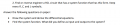I would imply I am allowed to use an active component in the circuit. Because the stuff you guys are talking about goes a little beyond my ability i thinkBut if you find a way to design this circuit without an opamp in it, I would be really interested to see your result!

Thanks for the help y'all!

#### MrAl

Joined Jun 17, 2014
10,620
Hello guys,

this is how the problem was stated in my textbook:

View attachment 270373

I would imply I am allowed to use an active component in the circuit. Because the stuff you guys are talking about goes a little beyond my ability i thinkBut if you find a way to design this circuit without an opamp in it, I would be really interested to see your result!

Thanks for the help y'all!
What is the system function, is it the same as before with the alpha, beta, and A, B, and C?

#### MrAl

Joined Jun 17, 2014
10,620
Thanks for that update. The actual roots of the quadratic term can be imaginary. I think what you meant was that the coefficients of the quadratic polynomial must be real and positive. Negative coefficients would potentially imply positive real parts which would lead to unstable behavior, and we just cannot tolerate THAT!
Hi,

Oh yes come to think of it maybe it is simpler because of that i'll have to double check.
I meant to try some other circuits too but got kind of loaded up with other stuff today maybe tomorrow afternoon.
It is interesting after all#### Fredje

Joined Jun 1, 2022
19
What is the system function, is it the same as before with the alpha, beta, and A, B, and C?
Yes, the one above is the system function

#### Papabravo

Joined Feb 24, 2006
20,404
Hi,

Oh yes come to think of it maybe it is simpler because of that i'll have to double check.
I meant to try some other circuits too but got kind of loaded up with other stuff today maybe tomorrow afternoon.
It is interesting after allYou can also rewrite the quadratic term with a coefficient of 1 for the squared term as:

$$s^2+\cfrac{B}{A}s+\cfrac{C}{A}\;=\;0$$

and the roots will be the same which can easily be demonstrated by the quadratic formula

#### Papabravo

Joined Feb 24, 2006
20,404

#### Fredje

Joined Jun 1, 2022
19
Here is a simulation that will actually check that your implementation matches a given transfer function. I just need the component values that you computed and the values of the transfer function coefficients. You can give then to me as in your original post and I will fill in the correct values on the simulation to see the results.
I had to solve this question without any component values. I had to keep all R,C,L symbolic. So I don't really know what to give you.
This is the way I solved it, I used KCL at node Vx, because I assume an ideal opamp I can say Vn = Vp: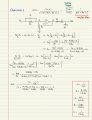#### Papabravo

Joined Feb 24, 2006
20,404
I chose the component values randomly and computed the values that should be in the transfer function. The problem is that the DC gain of your circuit is 40 dB lower than the DC gain of the transfer function. this suggests that you might want to take another look at the steps in the derivation. I'm not worried about the difference in response at high frequency because my "ideal" opamp is bandwidth limited and I can easily change it.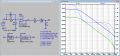R1​ R2​ R3​ C1​ C3​ L​ 10.00E+03​ 10.00E+03​ 10.00E+03​ 1.00E-06​ 1.00E-06​ 22.00E-06​ ​ ​ ​ ​ ​ ​ α​ 100​ ​ ​ ​ ​ β​ 200​ ​ ​ ​ ​ A​ 220.00E-15​ ​ ​ ​ ​ B​ 100.00E-06​ ​ ​ ​ ​ C​ 10.00E-03​ ​ ​ ​ ​